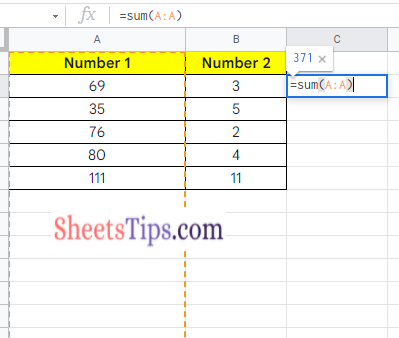# How to SUM a Column in Google Sheets? (Add Numbers, Row, Columns, Cells)

If you are a Google Sheets user, then you will be fond of adding rows and columns in between the cells as and when required. Most spreadsheet users will use Google Sheets to save the numbers and perform mathematical calculations. One of the widely used mathematical calculations in Google Sheets is adding a list of numbers in a column. We can easily add the numbers in columns using the SUM function in Google Sheets.

## How to SUM Cells in Google Sheets?

We can add two cells in Google Sheets either by using the SUM function or using the Addition operator. Let us discuss how to add two cells using the steps provided below:

• Step 2: Now in Cell 1 enter the number 1.
• Step 3: In Cell B, enter the number 2.
• Step 4: Now in Cell C, enter the formula =SUM(A2, B2).
• Step 5: Press the Return key and you will see the results.
• Step 6: Now drag the formula applied cells to other cells, to SUM other cells.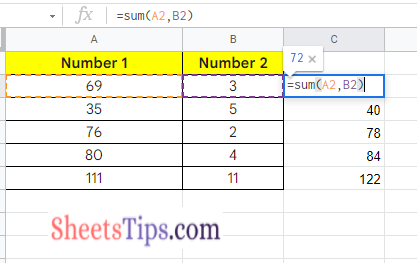The above steps explain to the user how to perform sum Cells using the SUM function. Alternatively, we can sum two cells using the addition operator and the steps to get this done are explained below:

• Step 2: Enter the 1st number in Cell A.
• Step 3: Enter the 2nd number in Cell B.
• Step 4: In Cell C, enter the formula =A2+B2 and press the Enter key.

You will see the results as shown in the image below.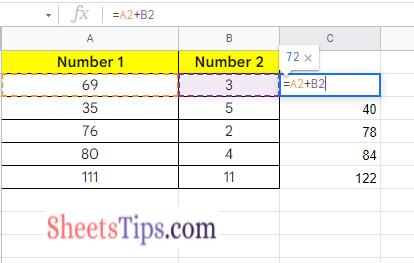### How to SUM Rows in Google Sheets?

In the above example, we saw how to SUM the columns in Google Sheets. Using the above we can even SUM the rows in Google Sheets. Here is an example of how to add two rows in Google Sheets.

• Step 1: Open the Google Sheets.
• Step 2: Enter the first number in Cell A2.
• Step 3: Enter the second number in Cell A3.
• Step 4: Now in the third row, i.e, in Cell A4, use the formula =A2+A3.
• Step 5: Press the “Enter” button and you will see the results as shown below.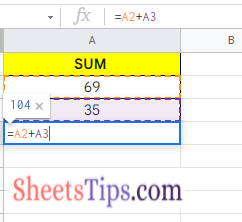We can also sum two rows using the SUM function in Google Sheets. The steps to SUM rows using the SUM function are explained below.

• Step 1: Open the Google Sheets.
• Step 2: Now move the Row 3 that is Cell A4.
• Step 3: Enter the formula =SUM(A2,A3).
• Step 4: Press the “Enter” key and you will see the results as shown in the image below.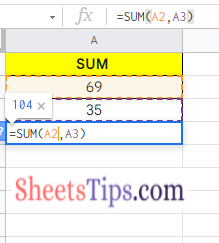### How to SUM Numbers in Google Sheets?

The steps to add two numbers in Google Sheets are as follows:

• Step 2: Now move to the cell where you want to add two numbers.
• Step 3: If you want to use SUM to function add number, then use the formula =SUM(Number 1, Number 2).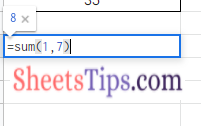Else move to step 4.

• Step 4: Enter the formula =number 1+number 2.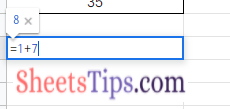• Step 5: Press the “Enter” key and you will see the results as shown in the image below.

### How to SUM a Column using SUM Function?

In some instances, we might fall under a situation where we want to SUM the entire column. The steps to get this done in Google Sheets are given below:

• Step 1: Open the Google Spreadsheet where you want to SUM column.
• Step 2: Now move to the cell where you want to SUM the entire column.
• Step 3: Simply enter the formula =SUM(A1, A10).
• Step 4: Press the “Enter” key and you will see the results.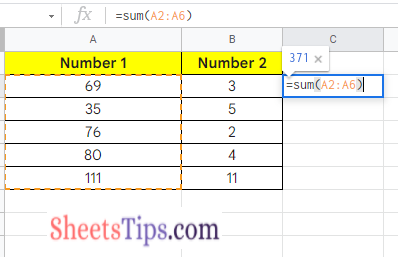### How to SUM Entire Column?

In the above example, we had to specify the cell range. However, if your data is expanded, then you might not get accurate results. And to overcome this issue, we can use the following steps.

• 1st Step: Open the Google Sheets.
• 2nd Step: Now move to cell where you want to get the SUM of an entire column.
• 3rd Step: Enter the formula =SUM(A: A).
• 4th Step: Press the “Return” key and you will see the results as shown below.